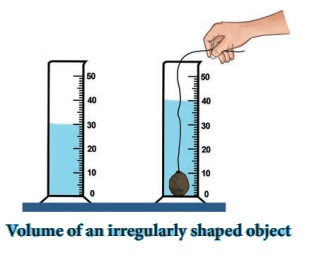Home | | Science 7th Std | Density

# Density

Take water in a beaker and drop an iron ball and a cork bowl into the water.

Density

Take water in a beaker and drop an iron ball and a cork bowl into the water. What do you observe? The iron ball sinks and the cork floats as shown in figure. Can you explain why? If your answer is “heavy objects sink in water and lighter objects float is water”, then, why does a metal coin sink in water whereas a much heavier wooden log floats? These questions can be answered when we understand the concept of density.ACTIVITY 3

Take a measuring cylinder and pour some water into it (Do not fill the cylinder completely). Note down the volume of water from the readings of the measuring cylinder. Take it as V1. Now take a small stone and tie it with a thread. Immerse the stone inside the water by holding the thread. This has to be done such that the stone does not touch the walls of the measuring cylinder (Figure). Now, the level of water has raised. Note down the volume of water and take it to be V2. The volume of the stone is equal to the raise in the volume of water.

V130CC ; V240 CC;

Volume of stone = V2 – V1

40 – 30 = 10 CC.From the activity 4, we observe that wooden block occupies more volume than the iron ball of same mass. Also, we observe that wooden block is lighter than the iron block of same size.

The lightness or heaviness of a body is due to density. If more mass is packed into the same volume, it has greater density. so , the iron block will have more mass than the wooden block of the same size. Therefore iron has more density.

Definition of density:

Density of a substance is defined as the mass of the substance contained in unit volume (1 m3).

If the mass of a substance is “M” whose volume is “V”, then, the equation for density is given as

Density (D) =  mass(M) / Volume(V )

D  = M / V

ACTIVITY 4

a. Take an iron block and a wooden block of same mass (say 1kg each). Measure their volume. Which one of them has more volume and occupies more volume?

b. Take an iron block and a wooden block of same size. Weigh them and measure their mass. Which one of them has more mass?

Unit of density

SI unit of density is kg/m3. The CGS unit of density is g/cm3.

Density of different materials

Different materials have different densities. The materials with higher density are called “denser” and the materials with lower density are called “rarer”.

The density of some widely used materials are listed in the following table 1.4.

Table 1.4 Density of some common substances, at room temperatureSuppose you have one Kg of iron and gold, which of them would have more volume than the other? Give your reason.

Problem 1.4

A solid cylinder of mass 280 kg has a volume of 4 m3. Find the density of cylinder.

Solution:

Density of cylinder              = mass of cylinder / volume of cylinder

=280/4 = 70 kg/m3

Problem 1.5

A box is made up of iron and it has a volume of 125 cm3. Find its mass. (Density of iron is 7.8 g / cm3).

Solultion:

Density               =Mass / Volume

Hence, Mass       =Volume × Density

=125 × 7.8 = 975 g.

Problem 1.6

A sphere is made from copper whose mass is 3000 kg. If the density of copper is 8900 kg/m3, find the volume of the sphere.

Solution:

Density = Mass / Volume

Hence, Volume = Mass / Density

= 3000 / 8900 = 30 / 89

=0.34 m3

The relationship between Mass, density and volume are represented in the following density triangle:* Density= Mass/ Volume

* Mass= Density × Volume

* Volume= Mass / Density

Relationship between density, mass and volume

Tags : Measurement | Term 1 Unit 1 | 7th Science , 7th Science : Term 1 Unit 1 : Measurement
Study Material, Lecturing Notes, Assignment, Reference, Wiki description explanation, brief detail
7th Science : Term 1 Unit 1 : Measurement : Density | Measurement | Term 1 Unit 1 | 7th Science